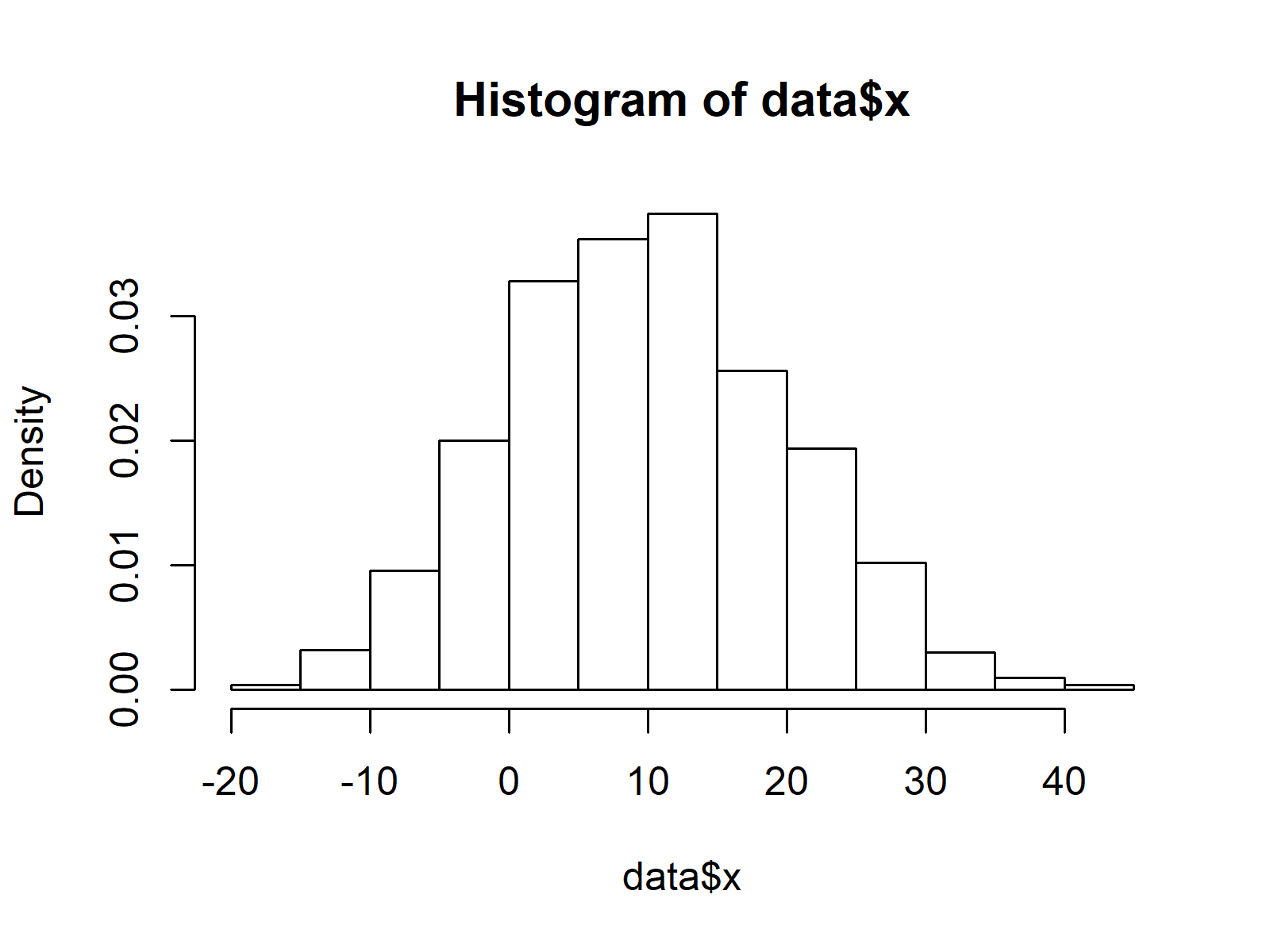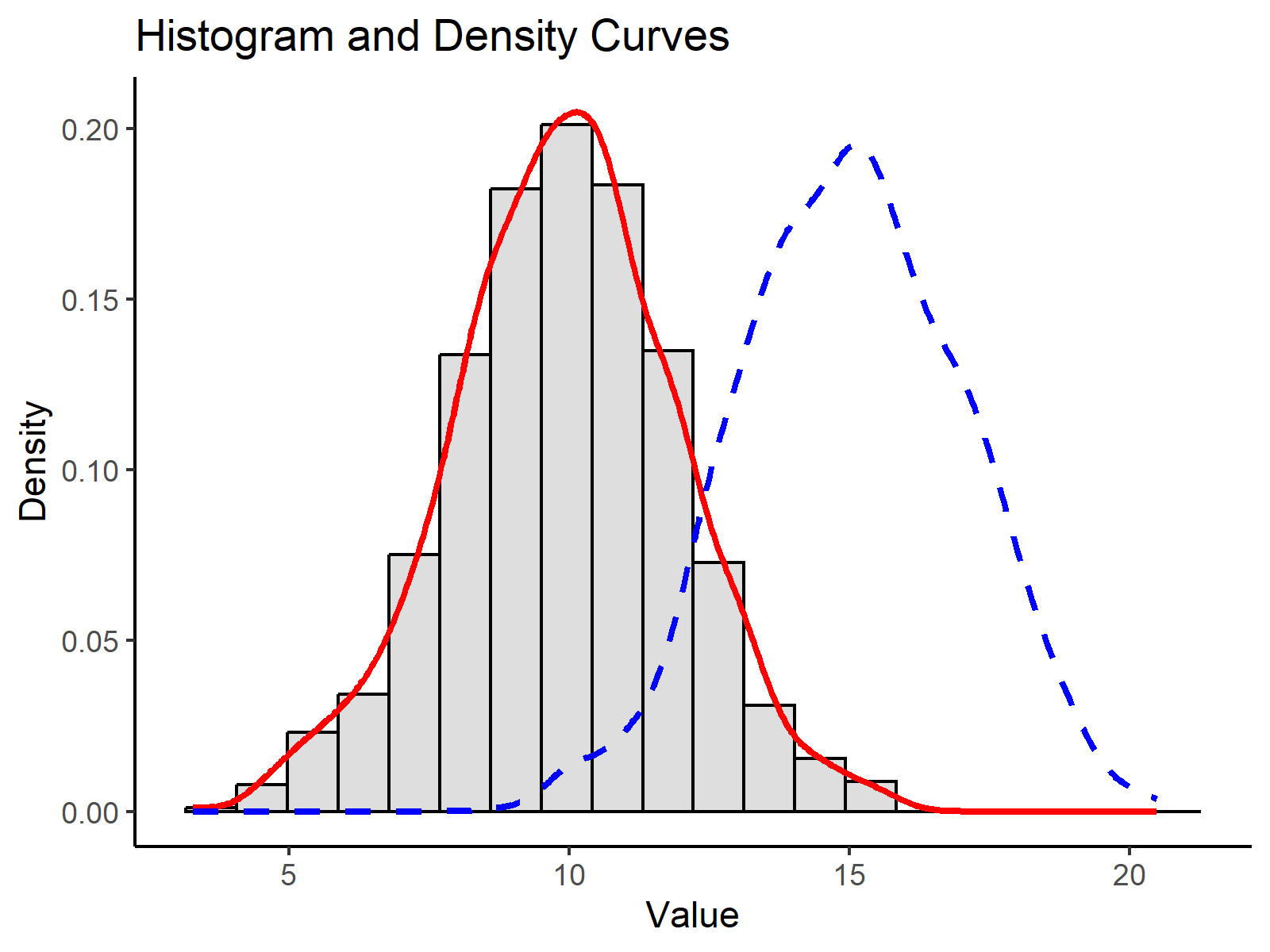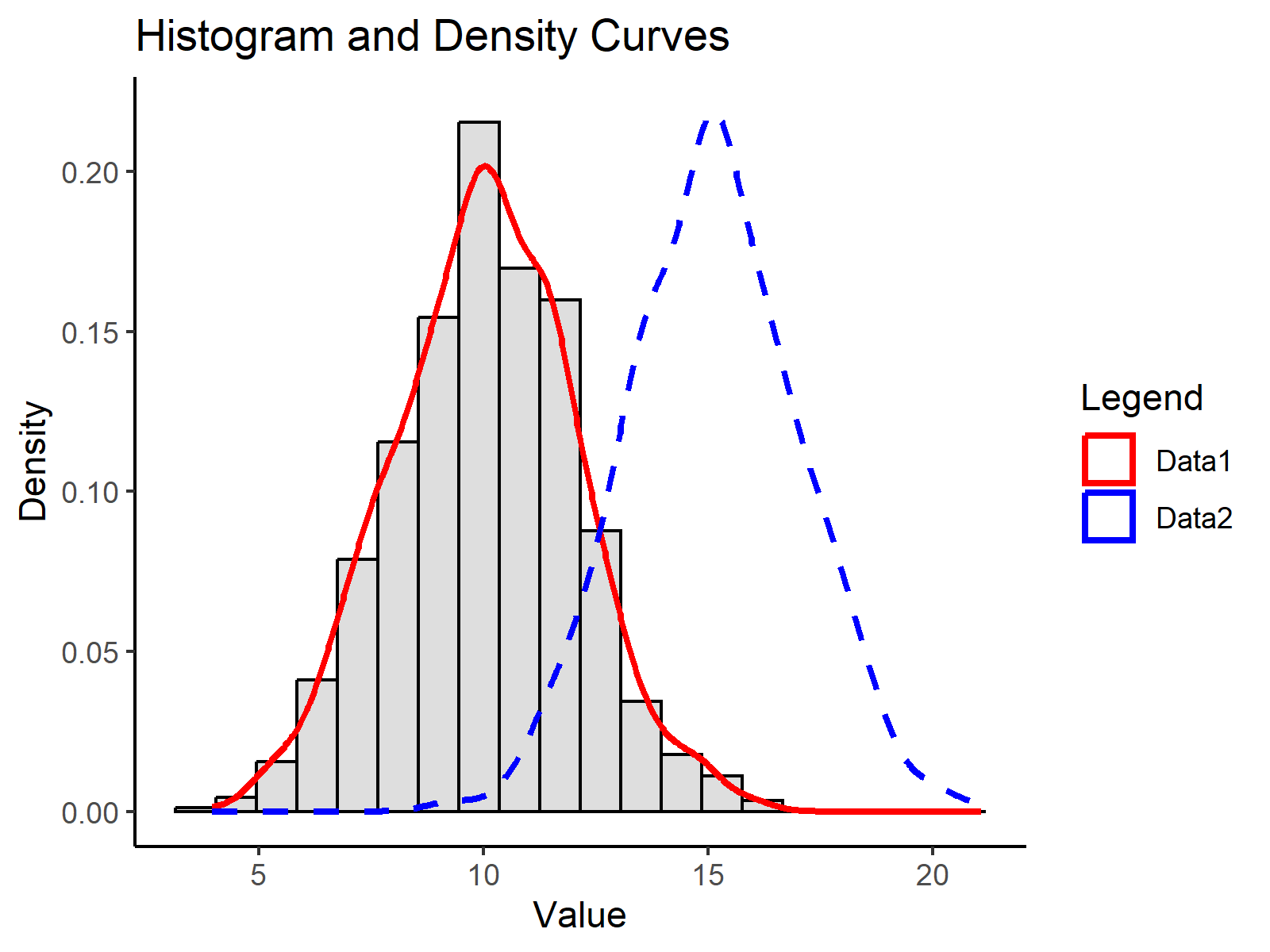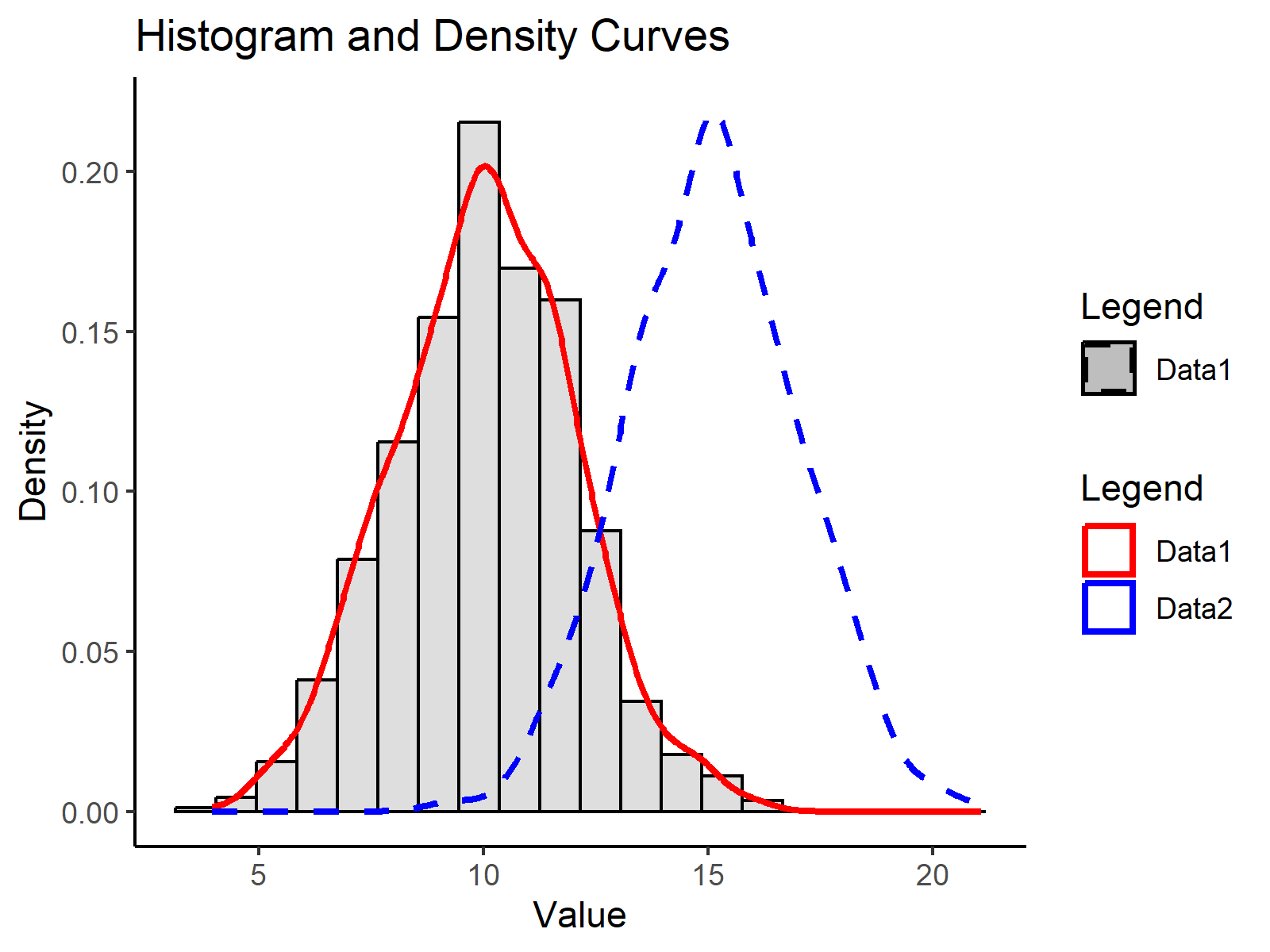# Overlay Histogram with Fitted Density Curve in Base R & ggplot2 Package (2 Examples)

In this tutorial you’ll learn how to fit a density plot to a histogram in the R programming language.

Let’s just jump right in!

## Introduction of Example Data

In the examples of this R programming tutorial, we’ll use the following example data:

```set.seed(18462)                                       # Create example data
data <- data.frame(x = round(rnorm(1000, 10, 10)))
#     x
# 1   6
# 2   7
# 3  14
# 4   4
# 5 -10
# 6  16```

As you can see based on the output of the RStudio console, our example data contains only one numeric column. Now, let’s draw these data

## Example 1: Histogram & Density with Base R

Example 1 explains how to fit a density curve to a histogram with the basic installation of the R programming language. First, we need to use the hist function to draw a histogram:

`hist(data\$x, prob = TRUE)                             # Create histogram with Base R`Figure 1: Histogram Created with Base R.

Figure 1 shows the output of the previous R code: A histogram without a density line. If we want to add a kernel density to this graph, we can use a combination of the lines and density functions:

`lines(density(data\$x), col = "red")                   # Overlay density curve`Figure 2: Histogram & Overlaid Density Plot Created with Base R.

Figure 2 illustrates the final result of Example 1: A histogram with a fitted density curve created in Base R.

## Example 2: Histogram & Density with ggplot2 Package

Example 2 shows how to create a histogram with a fitted density plot based on the ggplot2 add-on package. First, we need to install and load ggplot2 to R:

```install.packages("ggplot2")                           # Install & load ggplot2
library("ggplot2")```

Now, we can use a combination of the ggplot, geom_histogram, and geom_density functions to create out graphic:

```ggplot(data, aes(x)) +                                # ggplot2 histogram & density
geom_histogram(aes(y = stat(density))) +
geom_density(col = "red")```Figure 3: Histogram & Overlaid Density Plot Created with ggplot2 Package.

Figure 3 visualizes our histogram and density line created with the ggplot2 package. Note that the histogram bars of Example 1 and Example 2 look slightly different, since by default the ggplot2 packages uses a different width of the bars compared to Base R.

## Video, Further Resources & Summary

Some time ago I have published a video on my YouTube channel, which illustrates the content of this tutorial. You can find the video below:

Furthermore, you might want to have a look at some of the related articles which I have published on my homepage.

In this tutorial, I illustrated how to combine histograms with probability on the y-axis and density plots in the R programming language. If you have additional questions or comments, let me know in the comments section below.

Subscribe to the Statistics Globe Newsletter

•Ardy
December 19, 2022 6:58 am

Hello Joachim

Thanks for your nice videos. I have the following R script which is for only one .tsv file. I want to tweak it in a way that can plot (Histogram + line) two similar but separate .tsv files with different colours overlaid on each other. Could you please guide?

# filter Ks distribution (0.001 < Ks < 5)
lower_bound = 0.001
upper_bound = 5
df = df[df\$Ks lower_bound,]

# perform node-averaging (redo when applying other filters)
dff = aggregate(df\$Ks, list(df\$Family, df\$Node), mean)

# reflect the data around the lower Ks bound to account for boundary effects
ks = c(dff\$x, -dff\$x + lower_bound)

# plot a histogram and KDE on top
hist(ks, prob=TRUE, xlim=c(0, upper_bound), n=50)
lines(density(ks), xlim=c(0, upper_bound))

•December 19, 2022 4:09 pm

Hello Ardy,

Thank you for following us! Would you like to draw two density lines overlaid on each other on a histogram?

Regards,
Cansu

•Ardy
December 19, 2022 9:05 pm

Hello Cansu
Yes that is right.
Regards
Ardy

•December 20, 2022 10:25 am

Hello Ardy,

Here is how to do it in two ways (via graphics and ggplot2 libraries):

Expanding the data frame for this example:

```set.seed(18462)                                       # Create example data
data <- data.frame(x = round(rnorm(1000, 10, 10)), y= round(rnorm(1000, 20, 10)))

Using R graphics:

```hist(data\$x, prob = TRUE)
lines(density(data\$x), col = "red")
lines(density(data\$y), col = "blue")```

Using ggplot2 library:

```ggplot(data, aes(x)) +                                # ggplot2 histogram & density
geom_histogram(aes(y = stat(density))) +
geom_density(data=data, aes(x=x, y=stat(density)), col = "red") +
geom_density(data=data, aes(x=y, y=stat(density)), col = "blue")```

Regards,
Cansu

•Ardy
December 20, 2022 10:39 am

Dear Cansu
Thanks a lot for your help. Sorry I am so new to R. Could you pls let me know how/where to fit these codes in the contest of the following, if possible?

# filter Ks distribution (0.001 < Ks < 5)
lower_bound = 0.001
upper_bound = 5
df = df[df\$Ks lower_bound,]

# perform node-averaging (redo when applying other filters)
dff = aggregate(df\$Ks, list(df\$Family, df\$Node), mean)

# reflect the data around the lower Ks bound to account for boundary effects
ks = c(dff\$x, -dff\$x + lower_bound)

# plot a histogram and KDE on top
hist(ks, prob=TRUE, xlim=c(0, upper_bound), n=50)
lines(density(ks), xlim=c(0, upper_bound))

•Ardy
December 20, 2022 10:48 am

Sorry I am not sure about term overlay! But better to say overlap two graphs for comparison purpose.

•December 20, 2022 10:58 am

Hello again:)

Could we say that you would like to plot multiple histograms on the same panel? Not fitting multiple curves?

Regards,
Cansu

•Ardy
December 20, 2022 11:09 am

Yes, that is correct.

•md3948869
April 17, 2023 1:26 am

Thanks for your excellent website. I tried to draw a histogram with two densities using the following code.

ggplot(data, aes(x)) + # ggplot2 histogram & density
geom_histogram(aes(y = stat(density))) +
geom_density(data=data, aes(x=x, y=stat(density)), col = “red”) +
geom_density(data=data, aes(x=y, y=stat(density)), col = “blue”)
But the code doesn’t work.
I also tried to add a legend to my graph. but I couldn’t. For example.
set.seed(18462) # Create example data
data <- data.frame(x = round(rnorm(1000, 10, 10)))

p <- ggplot(data, aes(x)) + # ggplot2 histogram & density
geom_histogram(aes(y = stat(density))) +
geom_density(col = "red")
pal <- c("Observed"="black","Estimated"="blue")
pal
p <- p + scale_colour_manual(values = pal, limits = names(pal),
guide = guide_legend(reverse = TRUE))

But legend will not appear.

•April 18, 2023 2:47 pm

Hello,

Let’s first correct the plot gives error. You need two different data to plot two different density curves. I created 2 sample datasets for that data and data2. Then modify your code as follows:

```library(ggplot2)

data <- data.frame(var1 = rnorm(1000, 10, 2))
data2 <- data.frame(x = rnorm(1000, 15, 2))

ggplot(data, aes(x = var1)) +
geom_histogram(aes(y = ..density..), bins = 20, alpha = 0.5, color = "black", fill = "gray") +
geom_density(color = "red", size = 1) +
geom_density(data = data2, aes(x = x), color = "blue", linewidth = 1, linetype = "dashed") +
labs(title = "Histogram and Density Curves", x = "Value", y = "Density") +
theme_classic()```Please let me know if it works. Then try to add a legend. If it doesn’t work, let me know.

Regards,
Cansu

•md3948869
April 19, 2023 12:52 am

I’m sorry for again asking for help. The graph is OK, but I can’t add a legend to the graph.
p <- ggplot(data, aes(x = var1)) +
geom_histogram(aes(y = ..density..), bins = 20, alpha = 0.5, color = "black", fill = "gray") +
geom_density(color = "red", size = 1) +
geom_density(data = data2, aes(x = x), color = "blue", linewidth = 1, linetype = "dashed") +
labs(title = "Histogram and Density Curves", x = "Value", y = "Density")
p<- p + scale_colour_manual(name="Legend", values = c("hist" = "black", "data" = "red", "data2" = "blue"))
p

•April 19, 2023 9:00 am

Hello,

I have renamed the data for better visualization as follows.

```data <- data.frame(Data1 = rnorm(1000, 10, 2))
data2 <- data.frame(x = rnorm(1000, 15, 2))```

You can use the following script to specify the densities in the legend.

```ggplot(data, aes(x = Data1)) +
geom_histogram(aes(y = ..density..), bins = 20, alpha = 0.5, color = "black", fill = "gray") +
geom_density(aes(color = "Data1"), size = 1) +
geom_density(data = data2, aes(x = x, color = "Data2"), linewidth = 1, linetype = "dashed") +
scale_color_manual(name = "Legend", values = c("red", "blue"), labels = c("Data1", "Data2")) +
labs(title = "Histogram and Density Curves", x = "Value", y = "Density") +
theme_classic()```I see that you also want to specify the histogram. I am unsure how necessary it is since the red curve already represents the same data as the histogram. However, you can use something like this.

```ggplot(data, aes(x = Data1)) +
geom_histogram(aes(y = ..density.., fill = "Data1"), bins = 20, alpha = 0.5, color = "black") +
geom_density(aes(color = "Data1"), size = 1) +
geom_density(data = data2, aes(x = x, color = "Data2"), linewidth = 1, linetype = "dashed") +
scale_color_manual(name = "Legend", values = c("red", "blue"), labels = c("Data1", "Data2")) +
scale_fill_manual(name = "Legend", values = c("gray"), labels = c("Data1")) +
labs(title = "Histogram and Density Curves", x = "Value", y = "Density") +
theme_classic()```Regards,
Cansu

•md3948869
April 19, 2023 10:14 am

Thanks Cansu.
I want to be a statistician like you !!!!

•April 19, 2023 10:30 am

I am sure you will be.

Have a good one!
Cansu

Top